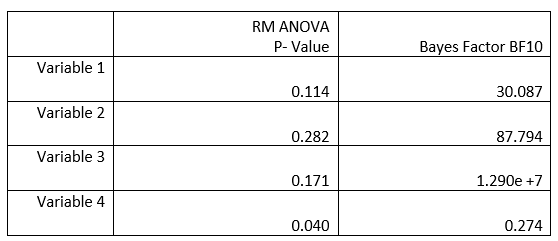#### Howdy, Stranger!

It looks like you're new here. If you want to get involved, click one of these buttons!

Supported by

# Bayesian RM ANOVA - Large Bayes Factor

Hi,

I have conducted RM ANOVA for my study with 2 groups and 4 conditions. 3 are insignificant where as 1 is significant. Therefore, I have decided to apply Bayesian RM ANOVA on the data. Given I’m new to Bayesian Statistics, I’m hoping to seek some guidance to ensure that I’m on the right track. Below is the p-value vs Bayes Factor:My questions are:
1. Bayes Factor for Variable 3 is extremely large compared to Variable 1 & 2. Is this unusual? What is the reason behind such a large Bayes Factor
2. Variable 4 has a significant p-value but no evidence in Bayes Factor. What is the explanation behind this?

Thanks for your help.

N_C

Here is the output from JASP

## Comments

• Hi N_C,

OK, so let's take variable 1. Going by the p=.114 value, you are interested in the timegroup interaction. If you look at the Bayesian ANOVA, you can see that the model with both main effects outpredicts the null model by a factor of 30, and that the model that also includes the interaction outpredicts the null model by a factor of 18. So the Bayes factor *against adding the interaction (compared to the two main effects models) is 30/18 = 1.7. Some other information is available on this forum, and in this paper: https://link.springer.com/article/10.3758/s13423-017-1323-7

Cheers,
E.J.

Thanked by 1N_C
Sign In or Register to comment.